## Air at 30°C and 2 MPa flows at steady state in a horizontal pipeline with a velocity of 25 m/s. It passes through a throttle valve where the

Question

Air at 30°C and 2 MPa flows at steady state in a horizontal pipeline with a velocity of 25 m/s. It passes through a throttle valve where the pressure is reduced to 0.3 MPa. The pipe is the same diameter upstream and downstream of the valve. What is the outlet temperature and velocity of the gas

in progress 0
2 weeks 2021-08-29T19:14:06+00:00 1 Answers 0 views 0

The outlet velocity is 159.9m/s

The outlet temperature = 290.6K

Explanation:

At initial condition

P=2 MPa

T=30°C

V=25 m/s

At final condition

P=0.3 MPa

Now from first law for open system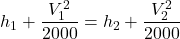We know that for air

h= 1.010 x T  KJ/kg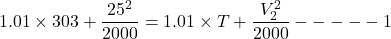Now from mass balance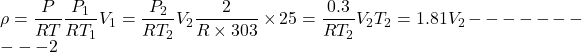Now from equation 1 and 2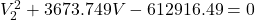So we can say that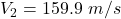The outlet velocity is 159.9m/s

Now by putting the values in equation 2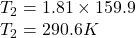The outlet temperature = 290.6K